# MCAT Physical : Resistors and Resistance

## Example Questions

2 Next →

### Example Question #21 : Circuits

During the cold winter months, some gloves have the ability to provide extra warmth due to an internal heating source. A simplified circuit, similar to those in electric gloves, is comprised of a 9 V battery with no internal resistance and three resistors as shown in the image below.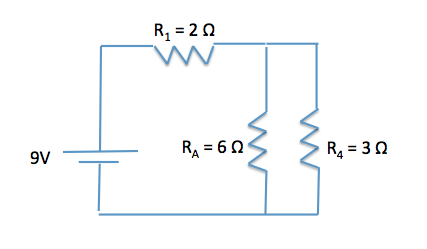All resistors in series share the same __________, while all resistors in parallel share the same __________.

potential energy . . . voltage

current . . . potential energy

current . . . voltage

voltage . . . current

current . . . voltage

Explanation:

As a common rule to all circuits, all resistors in series share the same current (due to conservation of charge) and all resistors in parallel share the same voltage. This information is very helpful in determining the voltage drops across sets of resistors and the current that flows through them.

### Example Question #51 : Ap Physics 2

What is the internal resistance of a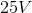battery that has a potential of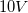when a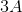current runs through it?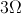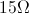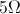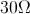Explanation:

The equation for a battery with internal resistance is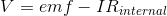Using this equation, we can solve for the resistance.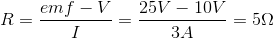### Example Question #61 : Electricity And Magnetismbattery is connected to a circuit which contains two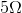resistors connected in parallel. What is the current throughout the circuit?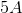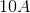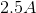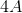Explanation:

We know the total voltage in the circuit is. We need to determine the total resistance within the circuit. Since we know there are two resistors connected in parallel we use the following formula: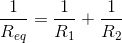Two resistors with resistances ofeach: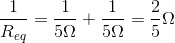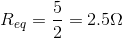Now we use another formula to determine the current using voltage and resistance: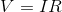Rearranged to:2 Next →

### All MCAT Physical Resources